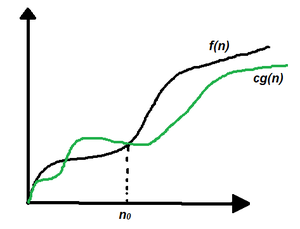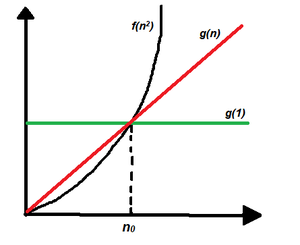# Analysis of Algorithms | Big – Ω (Big- Omega) Notation

In the analysis of algorithms, asymptotic notations are used to evaluate the performance of an algorithm, in its best cases and worst cases. This article will discuss Big – Omega Notation represented by a Greek letter (Ω).

Definition: Let g and f be the function from the set of natural numbers to itself. The function f is said to be Ω(g), if there is a constant c > 0 and a natural number n0 such that c*g(n) ≤ f(n) for all n ≥ n0

Mathematical Representation:

Ω(g) = {f(n): there exist positive constants c and n0 such that 0 ≤ c*g(n) ≤ f(n) for all n ≥ n0
Note: Ω (g) is a set

Graphical Representation:Graphical Representation

In simple language, Big – Omega (Ω) notation specifies the asymptotic (at the extreme) lower bound for a function f(n).

Follow the steps below to calculate Big – Omega (Ω) for any program:

1. Break the program into smaller segments.
2. Find the number of operations performed for each segment(in terms of the input size) assuming the given input is such that the program takes the least amount of time.
3. Add up all the operations and simplify it, let’s say it is f(n).
4. Remove all the constants and choose the term having the least order or any other function which is always less than f(n) when n tends to infinity, let say it is g(n) then, Big – Omega (Ω) of f(n) is Ω(g(n)).

Example: Consider an example to print all the possible pairs of an array. The idea is to run two nested loops to generate all the possible pairs of the given array.

The pseudo-code is as follows:

`int print(int a[], int n){    for (int i = 0; i < n; i++)     {        for (int j = 0; j < n; j++)        {            if(i != j)                cout << a[i] << " "                      << a[j] << "\n";        }    }}`

Below is the implementation of the above approach:

## C++

 `// C++ program for the above approach` `#include ` `using` `namespace` `std;`   `// Function to print all possible pairs` `int` `print(``int` `a[], ``int` `n)` `{` `    ``for` `(``int` `i = 0; i < n; i++) {` `        ``for` `(``int` `j = 0; j < n; j++) {` `            ``if` `(i != j)` `                ``cout << a[i] << ``" "` `<< a[j] << ``"\n"``;` `        ``}` `    ``}` `}`   `// Driver Code` `int` `main()` `{`   `    ``// Given array` `    ``int` `a[] = { 1, 2, 3 };`   `    ``// Store the size of the array` `    ``int` `n = ``sizeof``(a) / ``sizeof``(a);`   `    ``// Function Call` `    ``print(a, n);`   `    ``return` `0;` `}`

## Java

 `// Java program for the above approach` `import` `java.lang.*;` `import` `java.util.*;`   `class` `GFG{`   `// Function to print all possible pairs` `static` `void` `print(``int` `a[], ``int` `n)` `{` `    ``for``(``int` `i = ``0``; i < n; i++) ` `    ``{` `        ``for``(``int` `j = ``0``; j < n; j++) ` `        ``{` `            ``if` `(i != j)` `                ``System.out.println(a[i] + ``" "` `+ a[j]);` `        ``}` `    ``}` `}`   `// Driver code` `public` `static` `void` `main(String[] args)` `{` `    `  `    ``// Given array` `    ``int` `a[] = { ``1``, ``2``, ``3` `};`   `    ``// Store the size of the array` `    ``int` `n = a.length;`   `    ``// Function Call` `    ``print(a, n);` `}` `}`   `// This code is contributed by avijitmondal1998`

## Python3

 `# Python3 program for the above approach`   `# Function to print all possible pairs` `def` `printt(a, n) :` `    `  `    ``for` `i ``in` `range``(n) :` `        ``for` `j ``in` `range``(n) :` `            ``if` `(i !``=` `j) :` `                ``print``(a[i], "", a[j])`   `# Driver Code`   `# Given array` `a ``=` `[ ``1``, ``2``, ``3` `]`   `# Store the size of the array` `n ``=` `len``(a) `   `# Function Call` `printt(a, n)`   `# This code is contributed by splevel62.`

## C#

 `// C# program for above approach` `using` `System;`   `class` `GFG{`     `// Function to print all possible pairs` `static` `void` `print(``int``[] a, ``int` `n)` `{` `    ``for``(``int` `i = 0; i < n; i++) ` `    ``{` `        ``for``(``int` `j = 0; j < n; j++) ` `        ``{` `            ``if` `(i != j)` `                ``Console.WriteLine(a[i] + ``" "` `+ a[j]);` `        ``}` `    ``}` `}`     `// Driver Code` `static` `void` `Main()` `{` `     ``// Given array` `    ``int``[] a = { 1, 2, 3 };`   `    ``// Store the size of the array` `    ``int` `n = a.Length;`   `    ``// Function Call` `    ``print(a, n);` `}` `}`   `// This code is contributed by sanjoy_62.`

## Javascript

 ``

Output

```1 2
1 3
2 1
2 3
3 1
3 2
```

In this example, it is evident that the print statement gets executed n2 times therefore if the running time vs n graph is plotted a parabolic graph will be obtained, f(n2). Now linear functions g(n), logarithmic functions g(log n), constant functions g(1) all are less than a parabolic function when the input range tends to infinity therefore, the best-case running time of this program can be Ω(log n), Ω(n), Ω(1), or any function g(n) which is less than n2 when n tends to infinity. See the below graphical representation:When to use Big – Ω notation: Big – Ω notation is the least used notation for the analysis of algorithms because it can make a correct but imprecise statement over the performance of an algorithm. Suppose a person takes 100 minutes to complete a task, using Ω notation, it can be stated that the person takes more than 10 minutes to do the task, this statement is correct but not precise as it doesn’t mention the upper bound of the time taken. Similarly, using Ω notation we can say that the best-case running time for the binary search is Ω(1), which is true because we know that binary search would at least take constant time to execute.

For more details, please refer: Design and Analysis of Algorithms.

Feeling lost in the world of random DSA topics, wasting time without progress? It's time for a change! Join our DSA course, where we'll guide you on an exciting journey to master DSA efficiently and on schedule.
Ready to dive in? Explore our Free Demo Content and join our DSA course, trusted by over 100,000 geeks!

Previous
Next